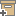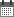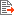ניהול
קהילה:
אסיף מאגר המחקר החקלאי
Individual Variation in Production Efficiency of Dairy and Beef Cows [abstract]Back to searchPrevious item
Next item
Year:
2005
Authors :
אהרוני, יואב
;
.
ברוש, אריה
;
.
Volume :
Co-Authors:
Facilitators :
From page:
244
To page:
244
(
Total pages:
1
)
Abstract:

Most of the metabolizable energy (MEI) consumed by cattle is lost as energy expended (EE) as heat. The energy balance of animals is presented by the following equation: MEI=EE+Retained Energy (RE). Knowledge of any two of the above variables allows the solution of the equation and calculation of energy efficiency. The objective of this presentation is to characterize the repeatability of differences in energy efficiency among cows, measured repeatedly in four experiments (Exp). The EE (kJ/(kgBW0.75*day)) in all experiments was measured by means of the heart rate (HR) method, i.e. HR that was measured throughout 3-4 days was multiplied by the oxygen consumption (VO2) per heartbeat, measured throughout 10-15 min, and by 20.47 kJ per liter VO2. In Exp 1, EE and MEI were measured nine times in six beef cows in confinement during one year of their reproductive cycle. In Exp 2, EE and MEI were measured in six non-lactating, non-pregnant beef cows on six diets varying in ME content. In Exp 3, EE and MEI were measured in five grazing seasons on differing herbage quality in16 cows. The data were analyzed by the ANOVA procedure with correction by covariance to MEI, to analyze differences among cows on an equal MEI basis. The differences in EE among cows in all experiments were significant (P range from 0.006 to 0.037), with range of individual variation of 20% to 28% of the average EE. In Exp 4 we used 19 Holstein dairy cows. Their EE and the energy in their secreted milk were measured four times during four months. The predicted EE of these cows was calculated from their energy in milk and their BW, assuming 0.57 MJ/kgBW0.75 for maintenance and efficiency of 64% of ME for milk production, and was subtracted from their measured EE to calculate their residual EE (REE). Thus, an efficient cow has a negative REE (predicted EE higher than measured EE). The repeatability of the REE among cows was significant (P<0.001). The REE ranged from -17% to +13% of the measured EE, i.e., a range of 30%. We conclude that lower EE in relation to MEI or predicted EE is a repeatable trait that should be considered as a tool to select cows of higher energy efficiency.

Note:
Related Files :
beef cattle
cows
dairy cattle
Efficiency
Energy expenditure
heart rate
עוד תגיות
תוכן קשור
More details
DOI :
Article number:
0
Affiliations:
Database:
Publication Type:
מאמר מתוך כינוס
;
.
תקציר
;
.
Language:
אנגלית
Editors' remarks:
ID:
51941
Last updated date:
02/03/2022 17:27
Creation date:
30/11/2020 14:11Scientific Publication
Individual Variation in Production Efficiency of Dairy and Beef Cows [abstract]
Individual Variation in Production Efficiency of Dairy and Beef Cows

Most of the metabolizable energy (MEI) consumed by cattle is lost as energy expended (EE) as heat. The energy balance of animals is presented by the following equation: MEI=EE+Retained Energy (RE). Knowledge of any two of the above variables allows the solution of the equation and calculation of energy efficiency. The objective of this presentation is to characterize the repeatability of differences in energy efficiency among cows, measured repeatedly in four experiments (Exp). The EE (kJ/(kgBW0.75*day)) in all experiments was measured by means of the heart rate (HR) method, i.e. HR that was measured throughout 3-4 days was multiplied by the oxygen consumption (VO2) per heartbeat, measured throughout 10-15 min, and by 20.47 kJ per liter VO2. In Exp 1, EE and MEI were measured nine times in six beef cows in confinement during one year of their reproductive cycle. In Exp 2, EE and MEI were measured in six non-lactating, non-pregnant beef cows on six diets varying in ME content. In Exp 3, EE and MEI were measured in five grazing seasons on differing herbage quality in16 cows. The data were analyzed by the ANOVA procedure with correction by covariance to MEI, to analyze differences among cows on an equal MEI basis. The differences in EE among cows in all experiments were significant (P range from 0.006 to 0.037), with range of individual variation of 20% to 28% of the average EE. In Exp 4 we used 19 Holstein dairy cows. Their EE and the energy in their secreted milk were measured four times during four months. The predicted EE of these cows was calculated from their energy in milk and their BW, assuming 0.57 MJ/kgBW0.75 for maintenance and efficiency of 64% of ME for milk production, and was subtracted from their measured EE to calculate their residual EE (REE). Thus, an efficient cow has a negative REE (predicted EE higher than measured EE). The repeatability of the REE among cows was significant (P<0.001). The REE ranged from -17% to +13% of the measured EE, i.e., a range of 30%. We conclude that lower EE in relation to MEI or predicted EE is a repeatable trait that should be considered as a tool to select cows of higher energy efficiency.

Scientific Publication
You may also be interested in